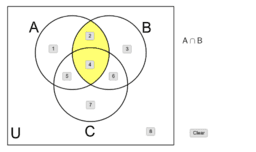# APS Geometry Unit 7 Book

Topic:
Geometry
MGSE9-12.S.CP.1 Describe categories of events as subsets of a sample space using unions, intersections, or complements of other events (or, and, not). MGSE9-12.S.CP.2 Understand that if two events A and B are independent, the probability of A and B occurring together is the product of their probabilities, and that if the probability of two events A and B occurring together is the product of their probabilities, the two events are independent. MGSE9-12.S.CP.3 Understand the conditional probability of A given B as P (A and B)/P(B). Interpret independence of A and B in terms of conditional probability; that is the conditional probability of A given B is the same as the probability of A and the conditional probability of B given A is the same as the probability of B. MGSE9-12.S.CP.4 Construct and interpret two-way frequency tables of data when two categories are associated with each object being classified. Use the two-way table as a sample space to decide if events are independent and to approximate conditional probabilities. For example, use collected data from a random sample of students in your school on their favorite subject among math, science, and English. Estimate the probability that a randomly selected student from your school will favor science given that the student is in tenth grade. Do the same for other subjects and compare the results. MGSE9-12.S.CP.5 Recognize and explain the concepts of conditional probability and independence in everyday language and everyday situations. For example, compare the chance of having lung cancer if you are a smoker with the chance of being a smoker if you have lung cancer. Use the rules of probability to compute probabilities of compound events in a uniform probability model.
• MGSE9-12.S.CP.6 Find the conditional probability of A given B as the fraction of B’s outcomes that also belong to A, and interpret the answer in context. MGSE9-12.S.CP.7 Apply the Addition Rule, P(A or B) = P(A) + P(B) – P(A and B), and interpret the answers in context.
•